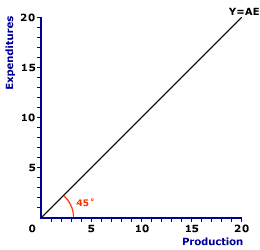Wednesday  February 8, 2023
 AmosWEB means Economics with a Touch of Whimsy!AFC: The abbreviation for average fixed cost, which is fixed cost per unit of output, found by dividing total fixed cost by the quantity of output. Average fixed cost is one of three related cost averages. The other two are average variable cost and avarage total cost. Average fixed cost decreases with larger quantities of output. Because fixed cost is FIXED and does not change with the quantity of output, a given cost is spread more thinly per unit as quantity increases. A thousand dollars of fixed cost averages out to \$10 per unit if only 100 units are produced. But if 10,000 units are produced, then the average shrinks to a mere 10 cents per unit.45-DEGREE LINE:

A line that shows equality between the variable measured on the vertical axis of a diagram and the variable measured on the horizontal axis. A 45-degree line is used both as a guideline that provides insight into the measured variables and as a critical part of the analysis. This line is perhaps most important in Keynesian economics. It is used in a diagram measuring consumption and income as a means of deriving saving---the difference between income and consumption. It is also used in the Keynesian model (or Keynesian cross) diagram measuring aggregate expenditures and aggregate production as a means of identifying equilibrium--the equality between aggregate expenditures and aggregate production.
45-Degree LineThe 45-degree line assists in the Keynesian economics evaluation and analysis of the macroeconomy. In general, a 45-degree line is so named because it forms a 45-degree angle with both the vertical or horizontal axes of a typical right-angle diagram. This is achieve because each point on the 45-degree line equates the variable measured on the vertical axis with the variable measured on the horizontal axis. Because the two variables are measured in the same units, the 45-degree line has a slope of one and it bisects the 90-degree angle formed by the vertical and horizontal axes.

A typical 45-degree line is presented in the exhibit to the right. In this particular exhibit, expenditures (aggregate expenditures) are measured on the vertical axis and production (aggregate production) is measured on the horizontal axis. The 45-degree line then depicts each point in this diagram in which aggregate production (Y) is equal to aggregate expenditures (AE). For this reason, the 45-degree line is also labeled Y=AE.

The two most common uses of the 45-degree line are the Keynesian model and the consumption line.

• Keynesian Model: The most important use of the 45-degree is the Keynesian model, which identifies equilibrium equality between aggregate expenditures and aggregate production. In particular, the intersection between the 45-degree line and the aggregate expenditures line indicates the equilibrium level of aggregate production.

• Consumption Line: The 45-degree line is also used as a guide for working with the consumption line. Because the 45-degree line has a slope of one, it serves to highlight that the slope of the consumption line is less that one. Moreover, because the 45-degree in this diagram shows the equality between consumption and income, it can be used to easily identify saving as the vertical difference between the consumption line an the 45-degree line.Recommended Citation:

45-DEGREE LINE, AmosWEB Encyclonomic WEB*pedia, http://www.AmosWEB.com, AmosWEB LLC, 2000-2023. [Accessed: February 8, 2023].

Check Out These Related Terms...

Or For A Little Background...

And For Further Study...
Search Again?PINK FADFLY[What's This?] Today, you are likely to spend a great deal of time surfing the Internet wanting to buy either a birthday gift for your grandfather or a pleather CD case. Be on the lookout for spoiled cheese hiding under your bed hatching conspiracies against humanity.Your Complete ScopeA half gallon milk jug holds about \$50 in pennies."Learn to enjoy every minute of your life. Be happy now. Don't wait for something outside of yourself to make you happy in the future. Think how really precious is the time you have to spend, whether it's at work or with your family. Every minuteshould be enjoyed and savored."-- Earl NightingaleTSPTime Series Econometrics (software)A PEDestrian's Guide Xtra CreditTell us what you think about AmosWEB. Like what you see? Have suggestions for improvements? Let us know. Click the User Feedback link.| | | | | | | | | | |
| | | |

Thanks for visiting AmosWEB